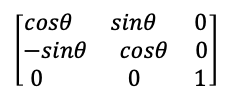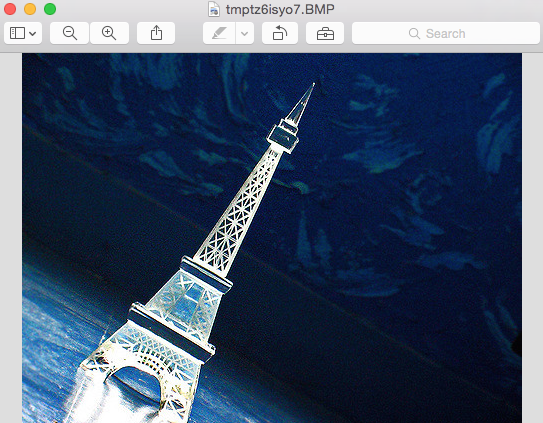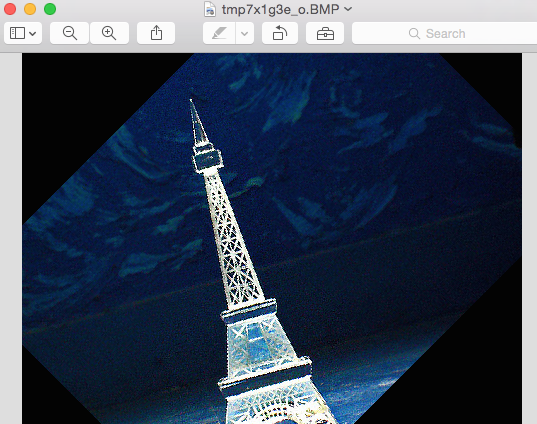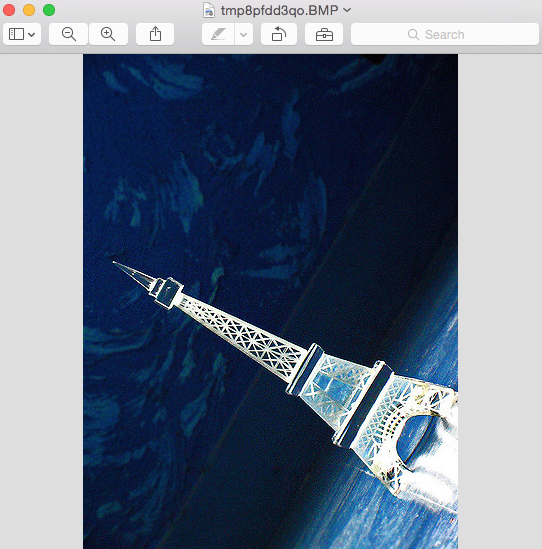# Rotate An Image Using Python Image Processing Library - Pillow

## Overview:

Rotation of an Image is one of the Image transform operations that can be applied on an Image. By Image rotation, the image is rotated about its center by specified number of degrees. Image rotation is a specialization of affine transformation.

Rotation is a geometric transformation. It can be done through either forward mapping or inverse mapping

The transformation function for forward mapping is x,y = T(v, w)  - x, y is the output pixel position and v, w is the input pixel position.

The transformation function for inverse mapping is v,w = T-1(x,y).

Pillow, the Python Image Processing library uses inverse mapping or reverse transformation. In inverse mapping, the input pixel positions are calculated using the output pixel positions. The output pixel values are calculated by applying one of the interpolation techniques on the neighborhood pixel values of the input pixel. The tranasformation matrix for the affine transformation in case of the rotate opeartion is given by:Where, θ is the angle of rotation.

Image rotation results in some pixel values beyond image boundaries, that is pixel values lying outside the dimension of the image. Such values will not be displayed in the output image shown. This happens when the number of degrees specified for Image rotation is not an integer multiple of 90 degrees.

## Rotation using Python and Pillow:

The rotate() method of Python Image Processing Library Pillow takes number of degrees as a parameter and rotates the  image in counter clockwise direction to the number of degrees specified.

Alternately, the transpose method can also be used with one of the constants Image.ROTATE_90, Image.ROTATE_180 and Image.ROTATE_270.

## Example:

 # import the Python Image processing Library from PIL import Image   # Create an Image object from an Image colorImage  = Image.open("./effil.jpg")   # Rotate it by 45 degrees rotated     = colorImage.rotate(45) # Rotate it by 90 degrees transposed  = colorImage.transpose(Image.ROTATE_90)   # Display the Original Image colorImage.show()   # Display the Image rotated by 45 degrees rotated.show()   # Display the Image rotated by 90 degrees transposed.show()

## Output: